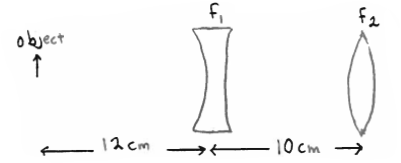# Problem: A diverging lens with focal length f1 = -20.0 cm is 10.0 cm to the left of a converging lens that has focal length f2 = +50.0 cm. An object that is 2.0 mm tall is placed 12.0 cm to the left of the diverging lens. The final image is formed after the light has passed through both lenses. How far is the final image from the converging lens?

###### Problem Details

A diverging lens with focal length f= -20.0 cm is 10.0 cm to the left of a converging lens that has focal length f= +50.0 cm. An object that is 2.0 mm tall is placed 12.0 cm to the left of the diverging lens. The final image is formed after the light has passed through both lenses. How far is the final image from the converging lens?